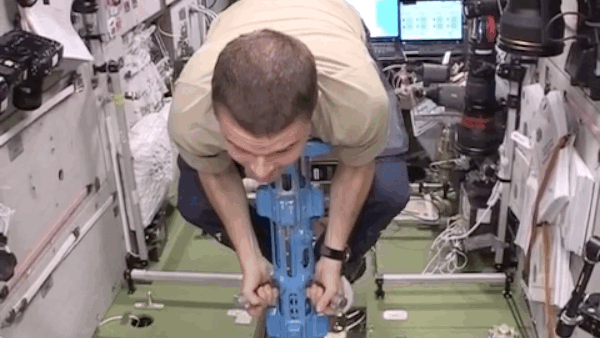## 20151122

### Physics quiz question: body mass measurement device

Physics 205A Quiz 6, fall semester 2015
Cuesta College, San Luis Obispo, CA"InsideISS #ISS #weight (Guess Reid Wiseman's Weight)"
space_station
vine.co/v/OKUDDvHgaJDAn astronaut (mass of 80.17 kg) aboard the International Space Station oscillates with a period of 0.93 s on a body mass measurement device. Approximate this as a mass-spring system (where the astronaut is the only mass attached to the spring), and ignore friction/drag. Take the gravitational constant g to be effectively zero aboard the International Space Station. The spring constant of the device is:
(A) 18 N/m.
(B) 58 N/m.
(C) 5.4×102 N/m.
(D) 3.7×103 N/m.

Correct answer (highlight to unhide): (D)

The period T of a mass m attached to a spring with spring constant k is given by:

T = 2·π·√(m/k),

such that the spring constant k will be:

k = m·(2·π/T)2 = (80.17 kg)·(2·π/(0.93 s))2 = 3,659.3649431 kg/s2,

or as expressed in more conventional units to two significant figures, the spring constant is 3.7×103 (kg·m/s2)·(1/m) = 170 N/m.

(Response (A) is 2·π·√(m/g); response (B) is 2·π·√(m/T); and response (C) is m·(2·π/T).)

Sections 70854, 70855, 73320
Exam code: quiz06m45S
(A) : 3 students
(B) : 6 students
(C) : 6 students
(D) : 56 students
(No response: 2 students)

Success level: 77%
Discrimination index (Aubrecht & Aubrecht, 1983): 0.52